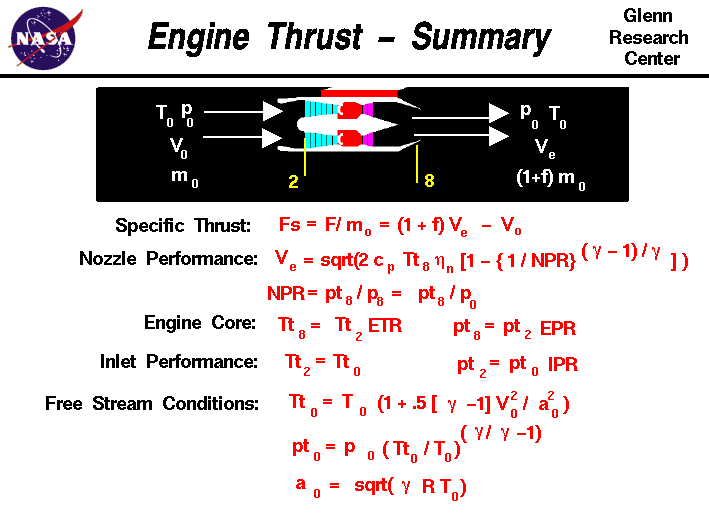+ Text Only Site
+ Non-Flash Version
+ Contact GlennOn this slide we have gathered together all of the equations necessary to compute the theoretical thrust for a turbojet engine. The general thrust equation is given just below the graphic in the specific thrust form. Fs = F / m = (1 + mf) * Ve - V0 where Fs is the specific thrust, F is the net thrust, m is the air flow rate through the engine, mf is the fuel-air ratio, Ve is the nozzle exit velocity, and V0 is the free stream velocity. The equation for the exit velocity was developed on the nozzle performance slide: Ve = sqrt( 2 * Cp * Tt8 * n8 * [1 - {1 / NPR} ^((gam - 1) / gam) ] ) where Cp is the specific heat at constant pressure, Tt8 is the total temperature in the nozzle, n8 is an efficiency factor, NPR is the nozzle pressure ratio, and gam is the ratio of specific heats. The NPR is simply the ratio of the total pressure in the nozzle pt8 to the free stream static pressure p0. NPR = pt8 / p0 The nozzle total pressure pt8 and the total temperature Tt8 depend on the engine pressure ratio EPR and temperature ratio. ETR. Tt8 = Tt2 * ETR pt8 = pt2 * EPR The equations for these ratios are given on separate slides and depend on the pressure and temperature ratio across each of the engine components. The engine pressure and temperature ratios are referenced to conditions at the compressor face, station 2. Compressor face conditions depend on free stream conditions and inlet performance, IPR: Tt2 = Tt0 pt2 = pt0 * IPR The equations at the bottom of the slide are compressible forms for determining the total pressure and temperature conditions from the corresponding static conditions and the free stream velocity. Tt0 = T0 * (1 + .5 * [gam -1] * V0 ^2 / a0 ^2) pt0 = p0 * (Tt0 / T0) ^ ( gam / ( gam -1)) where a0 is the free stream speed of sound. a0 = sqrt (gam * R * T0) where R is the gas constant from the equation of state. With the set of equations, knowing the free stream temperature, pressure and velocity, one can calculate the thrust generated by a engine design. These calculations are performed in the EngineSim engine simulator. A technical paper describing the details of the method used in EngineSim is also available. Activities:Guided ToursEngineSim - Engine Simulator:Thrust Equation:Navigation ..Beginner's Guide Home Page+ Inspector General Hotline + Equal Employment Opportunity Data Posted Pursuant to the No Fear Act + Budgets, Strategic Plans and Accountability Reports + Freedom of Information Act + The President's Management Agenda + NASA Privacy Statement, Disclaimer, and Accessibility CertificationEditor: Nancy Hall NASA Official: Nancy Hall Last Updated: May 13 2021 + Contact Glenn